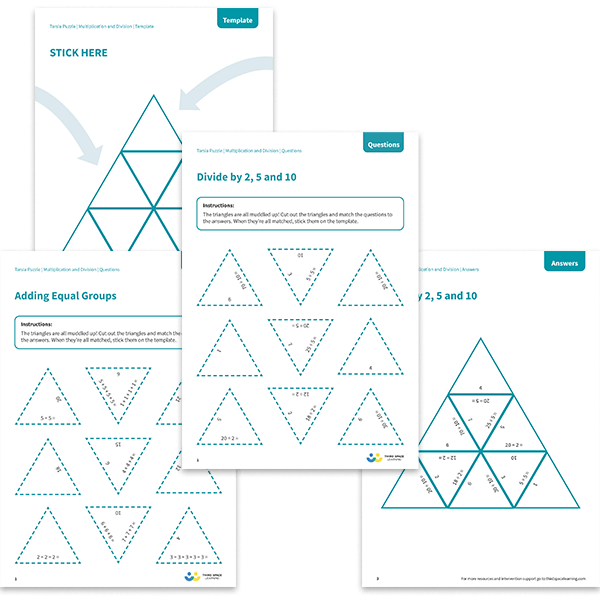# Tarsia Puzzles KS1 Multiplication and Division Pack

The triangles in the tarsia puzzle pack have all been mixed up. In these multiplication and division tarsia puzzles, pupils will need to match the question to the correct answer to create one large triangle.

This KS1 multiplication and division tarsia puzzle pack contains:

• One adding equal groups tarsia puzzle (Year 1 content)
• One doubles tarsia puzzle (Year 1 content)
• One sharing tarsia puzzle (Year 1 content)
• One multiplying by 2, 5 and 10 tarsia puzzle (Year 2 content)
• One dividing by 2, 5 and 10 tarsia puzzle (Year 2 content)

Each worksheet contains a set of questions, a template and an answer sheet.

Year 1, Year 2

Multiplication, Division